## Sunday, December 9, 2007

### Irodov Problem 1.109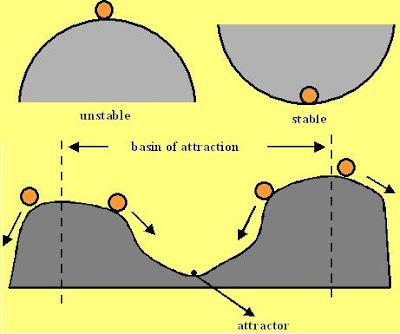First let us understand what is stability. Consider two balls, one kept at the bottom of a hemi-spherical bowl and the other kept on the top a hill. If we push the ball at the bottom of the bowl upwards a little bit, it will fall back into the bowl, oscillate for a while and then settle back at its original position. If however, we push the ball on the hill a little bit, it will immediately roll down the hill and never come back to the top. The ball in the bowl is stable since it has a tendency to revert back to its original state after being disturbed whereas the ball on the hill is unstable since it has a tendency to move away from its original position when disturbed.
Stability is essentially the tendency of something to get back to its original state even after being disturbed.

A system may be able to tolerate certain amounts of disturbance but may not be able to tolerate a very large disturbance. For example on the figure above, the stable point (also called the attractor) for the ball is the bottom of the valley. So far it is disturbed within the the two lines the ball will eventually revert back to the attractor however, if disturbed beyond the two lines it will not return back to the attractor. The region between the lines is called the basin of attraction for the attractor. In general there may be several basins of attractions and several attractors in a system.

How large a disturbance should an attractor be able to tolerate in order to be considered stable? This is where engineering and mathematics move apart. In mathematics, an attractor is stable if there it a basin of attraction even if the very very small one. In other words even there is a small neighborhood of stability the attractor is considered stable. The reason is in a subject as pure as mathematics, without any reference for comparison with some physical system, how can you define what is small? In engineering however, every system has a tolerance and the system is considered stable if it is stable within the specified tolerance requirements. In this problem we shall only consider the mathematical definition.Now let us come to the problem at hand. Consider that a particle is moving in a circular path in the force field as defined in the problem. When is it considered stable? If some sudden small disturbance moves it off-course and the particle has a tendency to revert back to its original circular path and not shoot out.

Let us start by understanding the nature of the circular orbit. Suppose that the velocity of particle is vo and it moves along a circular then its centripetal acceleration (centripetal force when view from the particle) must be equal to the acceleration induced by the force acting on it. In other words if the radius of the circle is ro and vo can be related as,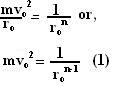The first thing to understand in this problem is that since the force is always acting perpendicular to the particle's velocity vector, it will never change the particle's magnitude of velocity vo (tangential component of velocity) but only influence its direction of motion ie. induce a rotation in the velocity vector. Why?

The velocity vector (v,) in polar coordinates can be represented in Cartesian coordinates as. The force acts perpendicular to this and can be written as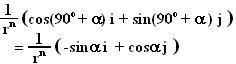Hence we have,This clearly shows the inability of the force to change the magnitude of velocity of the particle.
This is in principle not very different from a case where you tie a stone to a string and rotate it at constant speed. Here the tension in the string is the force acting on the stone and it always acts perpendicular to the velocity vector and so it never changes its speed but only changes its direction of motion.

Now let us see what happens if the particle is disturbed from its stable path. What are the kinds of disturbances that can occur? The disturbances can occur in the position vector (ro,) and velocity vector (vo ,) of the particle as shown in the figure. One this that simplifies thinking about the problem is the fact that while the particle rotates in a circular pathandkeep changing whoever,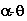(angle between velocity and radius vectors) is always 90 degrees. In other words i) r = ro and ii)represents the steady state of the particle moving in a circle. Disturbances in r,v andfrom their stable values ro , vo and 90 are then what matter to us.

First let us see the effect of a sudden change in velocity of the particle from vo to. We have already seen that the force itself cannot change the value of v as it always acts perpendicular to the vector. A disturbance in the magnitude of velocity v thus will never revert back to the original value for any value of n. In other words v will never return back to vo. How is it stable then? The particle might settle in a new circle with a new radius given by,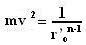Hereis the new radius that will the particle will settle in with the new velocity v. Essentially if the particle is stable after the disturbance it must converge to the new stable circle state r =and= 90 sometime after the disturbance.Given this it will be convenient to define disturbance in term of in terms of r andas,For stability we have to show that for small perturbationsandwill eventually die out.

Now let us consider the particle's motion in polar coordinates and suppose that the particle's coordinates at some instant are (r,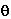). Let us resolve the velocity of the particle along the radial and the tangent to the radius vector as shown in the figure. Thus, we have,Equations (5) and (7) can now be rewritten in terms of disturbances as,Equations (8) and (9) now can be used to determine the stability of the circular orbit. The question to be asked is given (8) and (9) given an initial disturbance will the particle shoot off of the orbit or will it come back towards the original circle?

One straight-forward test of local stability can be obtained by linearizing (8) and (9) by considering only small disturbances. We can use Tailor's series to expand (8) and (9) and linearize it by neglecting the higher order terms with power greater or equal to 2. Hence we have,It is clear that Equations (12) and (13) both are equations of a simple harmonic oscillator when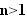, the oscillations will tend to diverge since the second derivatives always reinforce the displacement to increase. In other words if the circular path will never be stable and the particle will simply move away from the circular path. For small disturbances whenthe system will act like a simple harmonic oscillator and hence be stable. What about large disturbances when when we cannot linearize the system?
From (8) and (9) we get,From (4) it is clear that when, the second derivative will always act against the disturbanceand try and stabilize the system. However if during the course of evolution of the system if the disturbances inincrease beyond 90 degrees this will positively reinforce the disturbances and lead to instability. Further the functionis not symmetric about ro. For negative perturbations close to ro (+ ro close to 0) the value of acceleration will be very very large leading to a chaotic behavior. It is clear that the system of equations will not be stable beyond certain values of disturbances.

To determine these values we have to actually solve the either numerically or analytically. Perhaps there is an analytical solution but I took the easy way out and used Matlab (or octave) to solve them. The differential equations will be stiff (small numerical errors while numerically computing the equation can result in un-controlled error propagation) when+ ro close to 0 so its better to keep the values far away from this. What I found is that while the system is stable for large disturbances init is very sensitive to large disturbances in. The basin of attractionfor depends very much on n, m, initial value ofand v. For n=0.5, v=1,m=1 , I found numerically thatdegrees can make it unstable forgreater than 0.4 initially. Anyway I am sure further investigations can reveal more interesting facts.